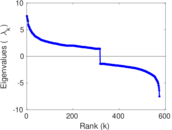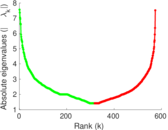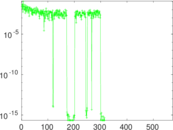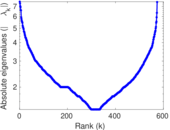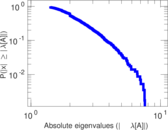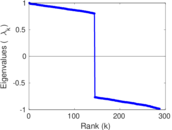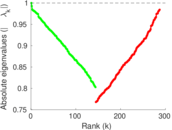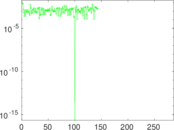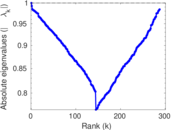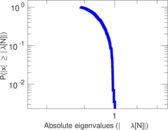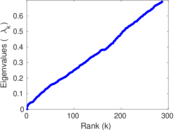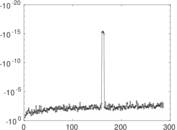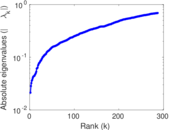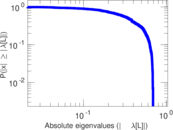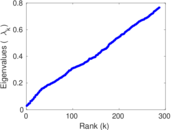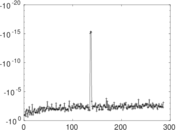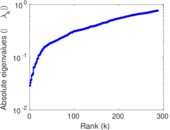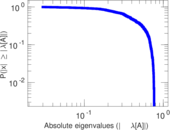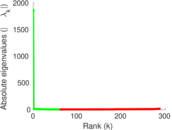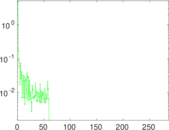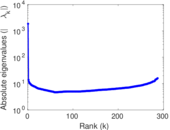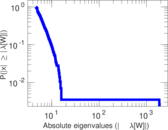# Yeast

This undirected network contains protein interactions contained in yeast. Research showed that proteins with a high degree were more important for the surivial of the yeast than others. A node represents a protein and an edge represents a metabolic interaction between two proteins. The network contains loops.

 Code `Mp` Internal name `moreno_propro` Name Yeast Data source http://moreno.ss.uci.edu/data.html#pro-pro AvailabilityDataset is available for download Consistency checkDataset passed all tests Category Metabolic network Node meaning Protein Edge meaning Interaction Network format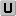Unipartite, undirected Edge typeUnweighted, no multiple edges Loops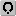Contains loops

## Statistics

 Size n = 1,870 Volume m = 2,277 Loop count l = 74 Wedge count s = 12,107 Claw count z = 75,064 Cross count x = 611,457 Triangle count t = 222 Square count q = 461 4-Tour count T4 = 56,522 Maximum degree dmax = 56 Average degree d = 2.435 29 Fill p = 0.001 301 60 Size of LCC N = 1,458 Diameter δ = 19 50-Percentile effective diameter δ0.5 = 6.390 63 90-Percentile effective diameter δ0.9 = 9.589 12 Median distance δM = 7 Mean distance δm = 7.069 74 Gini coefficient G = 0.449 043 Balanced inequality ratio P = 0.328 063 Relative edge distribution entropy Her = 0.943 033 Power law exponent γ = 2.869 77 Tail power law exponent γt = 3.041 00 Tail power law exponent with p γ3 = 3.041 00 p-value p = 0.611 000 Degree assortativity ρ = −0.161 530 Degree assortativity p-value pρ = 3.843 25 × 10−27 Clustering coefficient c = 0.055 009 5 Spectral norm α = 7.561 90 Algebraic connectivity a = 0.021 259 3 Spectral separation |λ1[A] / λ2[A]| = 1.006 17 Non-bipartivity bA = 0.006 130 51 Normalized non-bipartivity bN = 0.015 761 1 Algebraic non-bipartivity χ = 0.028 868 1 Spectral bipartite frustration bK = 0.002 639 85 Controllability C = 614 Relative controllability Cr = 0.328 342

## Plots

### Fruchterman–Reingold graph drawing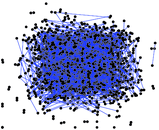### Degree distribution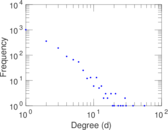### Cumulative degree distribution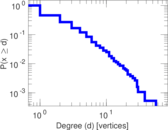### Lorenz curve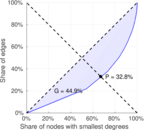### Spectral distribution of the adjacency matrix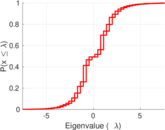### Spectral distribution of the normalized adjacency matrix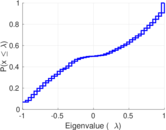### Spectral distribution of the Laplacian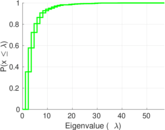### Spectral graph drawing based on the adjacency matrix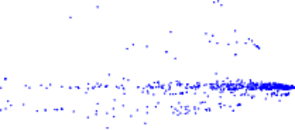### Spectral graph drawing based on the Laplacian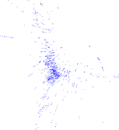### Spectral graph drawing based on the normalized adjacency matrix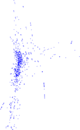### Degree assortativity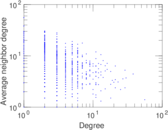### Zipf plot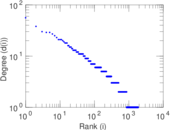### Hop distribution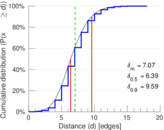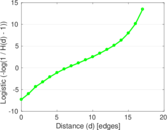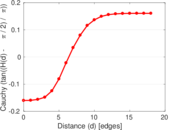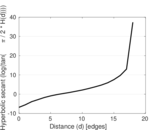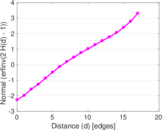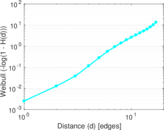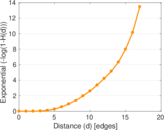### Double Laplacian graph drawing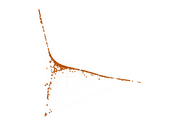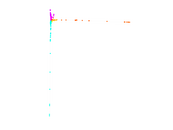### Delaunay graph drawing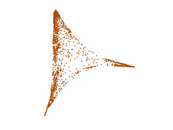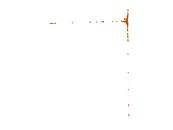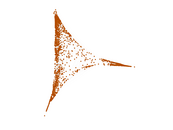### Clustering coefficient distribution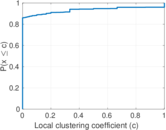### Average neighbor degree distribution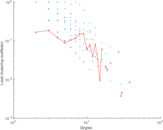### SynGraphy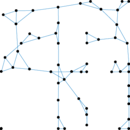### Matrix decompositions plots